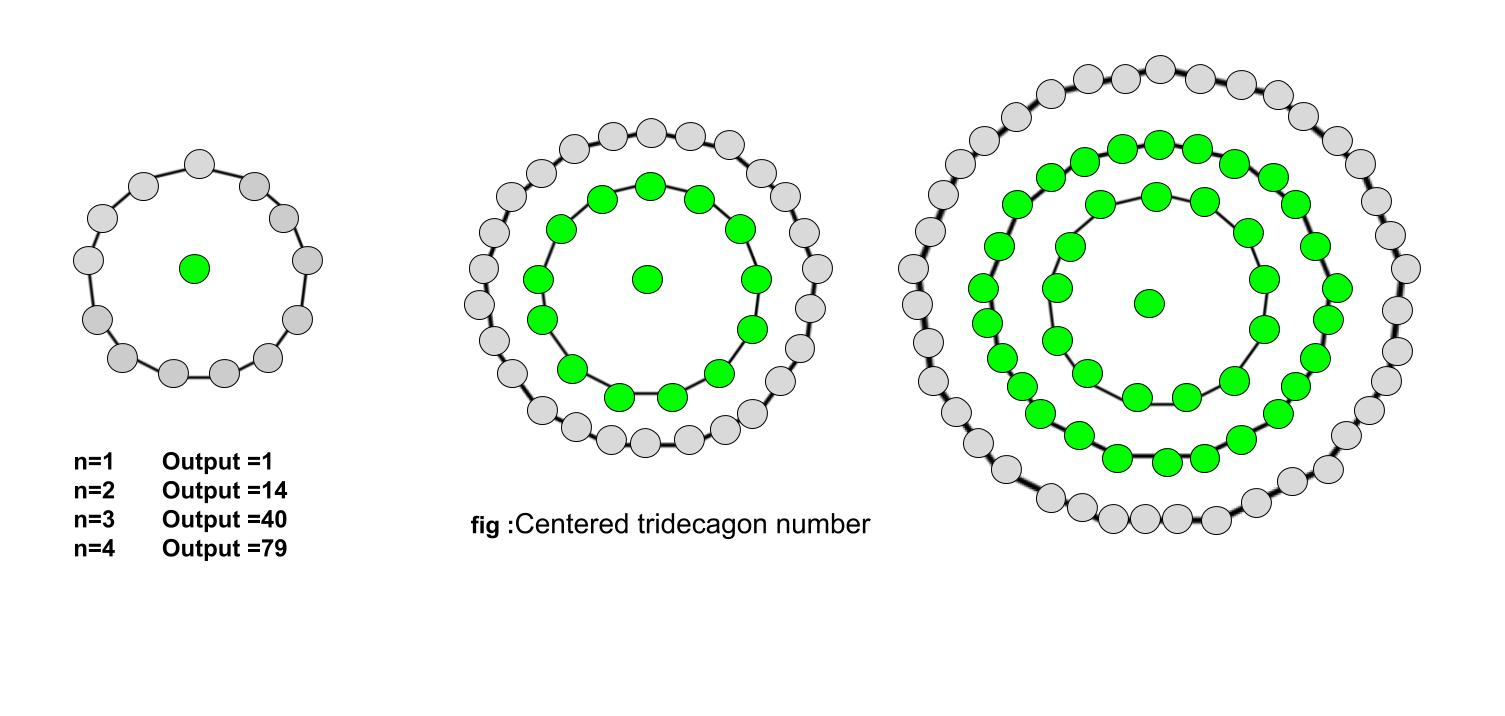# Centered tridecagonal number

Given a number n, the task is to find the nth Centered Tridecagonal Number.

A Centered tridecagonal number represents a dot at the center and other dots surrounding the center dot
in the successive tridecagonal(13 sided polygon) layer.

Examples :

Input : 2
Output : 14

Input : 9
Output : 469## Recommended: Please try your approach on {IDE} first, before moving on to the solution.

Formula for nth Centered tridecagonal number:

``````

## C++

 `// C++ Program to find nth ` `// centered tridecagonal number ` `#include ` `using` `namespace` `std; ` ` `  `// Function to find nth centered ` `// tridecagonal number ` `int` `centeredTridecagonalNum(``long` `int` `n) ` `{ ` `    ``// Formula to calculate nth ` `    ``// centered tridecagonal number ` `    ``return` `(13 * n * (n - 1) + 2) / 2; ` `} ` ` `  `// Drivers code ` `int` `main() ` `{ ` `    ``long` `int` `n = 3; ` `    ``cout << centeredTridecagonalNum(n); ` `    ``cout << endl; ` `    ``n = 10; ` `    ``cout << centeredTridecagonalNum(n); ` ` `  `    ``return` `0; ` `} `

## Java

 `// Java Program to find nth ` `// centered tridecagonal number ` `import` `java.io.*; ` ` `  `class` `GFG  ` `{ ` ` `  `// Function to find nth centered ` `// tridecagonal number ` `static` `long` `centeredTridecagonalNum(``long` `n) ` `{ ` `    ``// Formula to calculate nth ` `    ``// centered tridecagonal number ` `    ``return` `(``13` `* n * (n - ``1``) + ``2``) / ``2``; ` `} ` ` `  `// Driver Code ` `public` `static` `void` `main (String[] args)  ` `{ ` `    ``long` `n = ``3``; ` `    ``System.out.println(centeredTridecagonalNum(n)); ` `    ``n = ``10``; ` `    ``System.out.println(centeredTridecagonalNum(n)); ` `} ` `} ` ` `  `// This code is contributed by anuj_67. `

## Python3

 `# Program to find nth centered  ` `# tridecagonal number ` ` `  `# Function to find centered ` `# tridecagonal number ` `def` `centeredTridecagonalNum(n) : ` `     `  `    ``# Formula to calculate nth ` `    ``# centered tridecagonal number ` `    ``return` `(``13` `*` `n ``*`  `           ``(n ``-` `1``) ``+` `2``) ``/``/` `2` ` `  `# Driver Code ` `if` `__name__ ``=``=` `'__main__'` `: ` `         `  `    ``n ``=` `3` `    ``print``(centeredTridecagonalNum(n)) ` `    ``n ``=` `10` `    ``print``(centeredTridecagonalNum(n)) ` `                 `  `# This code is contributed ` `# by akt_mit `

## C#

 `// C# Program to find nth ` `// centered tridecagonal number ` `using` `System; ` ` `  `class` `GFG  ` `{ ` ` `  `// Function to find nth centered ` `// tridecagonal number ` `static` `long` `centeredTridecagonalNum(``long` `n) ` `{ ` `    ``// Formula to calculate nth ` `    ``// centered tridecagonal number ` `    ``return` `(13 * n * (n - 1) + 2) / 2; ` `} ` ` `  `// Driver Code ` `public` `static` `void` `Main ()  ` `{ ` `    ``long` `n = 3; ` `    ``Console.WriteLine(centeredTridecagonalNum(n)); ` `    ``n = 10; ` `    ``Console.WriteLine(centeredTridecagonalNum(n)); ` `} ` `} ` ` `  `// This code is contributed by anuj_67. `

## PHP

 ` `

Output :

```40
586
```

Reference: http://oeis.org/wiki/Figurate_numbers

My Personal Notes arrow_drop_upCheck out this Author's contributed articles.

If you like GeeksforGeeks and would like to contribute, you can also write an article using contribute.geeksforgeeks.org or mail your article to contribute@geeksforgeeks.org. See your article appearing on the GeeksforGeeks main page and help other Geeks.

Please Improve this article if you find anything incorrect by clicking on the "Improve Article" button below.

Improved By : vt_m, jit_t